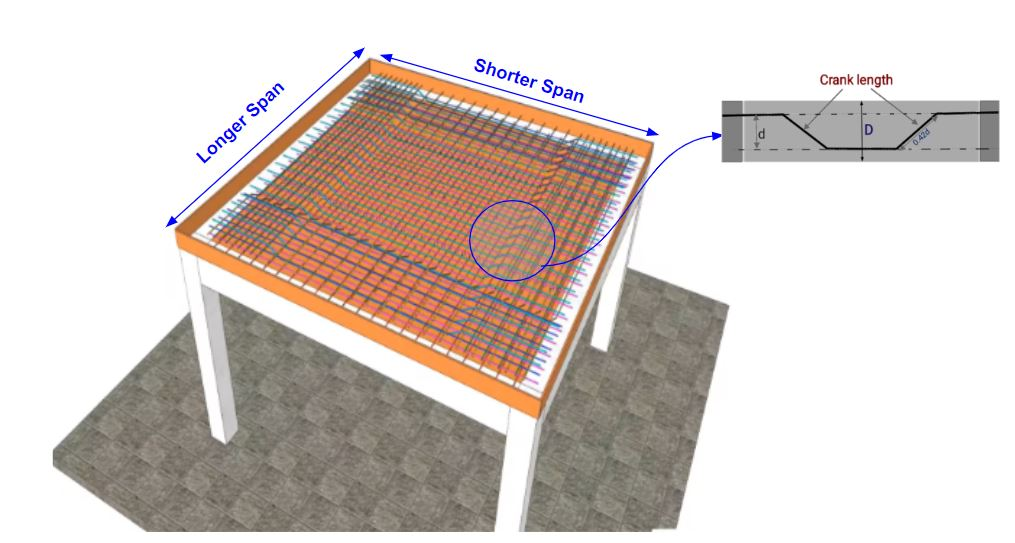Work Hours
Monday to Friday: 7AM - 7PM
Weekend: 10AM - 5PM

# Steel Weight Calculator for Two Way Slab

## Calculations for Steel Calculator for Two Way Slab

Let’s understand with the help of an example, how to use the above Steel Calculator for Two-Way Slab.Assumptions For Example

• There are 2 types of bars in two-way slab: Main Bar & Distribution Bar
• Main Bar Diameter = 10 mm
• Spacing Between Main Bars(C/C distance) = 150 mm
• Distribution Bar Diameter = 8 mm
• Spacing Between Distribution Bars(C/C distance) = 100 mm
• Length of Slab = 6000 mm
• Width of Slab = 3000 mm
• Width of Beam of Slab = 300 mm
• Concrete Cover = 25 mm
• Slab Thickness = 150 mm
• We are using a Steel grade of fe500

Calculations Procedure

• Step 1: Calculation of Bar Numbers
Calculate the number of bars required (main and distribution both).
Number of Main Bars = (Length of Longer Side / Spacing) + 1
=(( 6000/150)+1)
= 41
Number of Distribution Bars = (Length of Shorter Side / Spacing) + 1
= ((3000/100)+1)
= 31

• Step 2: Calculation of cutting length of main & distribution bar
Cutting Length of Main Bar = Clear Span (L) + (2 x Beam Width) – (2 x Con. Cover) + (1 x Inclined Length) – (45° bend x 2)
where L = Clear Span of the Slab ;0.42D = Inclined length (Bend length) ;1d = 45° bends (d is diameter of bar)
First calculate the length of “D“
D = (Thickness) – 2 (Clear cover at Top, Bottom) – Diameter of the bar
D = 150- 2×25 – 10
= 90 mm
Cutting Length of Main Bar = 3000 – 2×300 – 2×50 + 0.42×90 – 2×10
= 2318 mm
Cutting Length of Distribution Bar = Clear Span (L) + (2 x Beam Width) – (2 x Con. Cover) + (1 x Inclined Length) – (45° bend x 2)
where L = Clear Span of the Slab ;0.42D = Inclined length (Bend length) ;1d = 45° bends (d is diameter of bar)
Cutting Length of Distribution Bar = 6000 – 2×300 – 2×50 + 0.42×90 – 2×10
= 5318 mm

• Step 3:  Calculation of Top Distribution Bar
Numbers of Top bar for Longer span = [(L/5)/spacing]
= [(3000/5)/100]
= 6
Cutting Length of Top Bar for Longer span = Clear Span + (2 x Ld)
= 6000 + (2 x 40 x 8)
= 6640 mm
Numbers of Top bar for Shorter span = [(L/5)/spacing]
= [(6000/5)/150]
= 8
Cutting Length of Top Bar for Shorter span = Clear Span + (2 x Ld)
= 3000 + (2 x 40 x 10)
= 3800 mm

• Step 4: Total Length Calculation
Total Length of Main Bar = (41 x 2318) + (8 x 3800)
= 125438 mm
= 125.438 m = 126 m (approx)
Total Length of Distribution Bar = (31 x 5318) + (6 x 6640)
= 204698 mm
= 204.698 m = 205 m (approx)

• Step 5: Calculation of Steel Weight
Weight of the bar as per their length and diameter by following the Formula: –
Unit weight formula for kg per metre =(D2/163) × L kg/m
Unit weight for feet per metre = (D2/ 533) × L kg/ft
L is the required length of the Steel bar of Diameter D
Weight of Main Bar = (102/163) x 126
= 77.3 Kg
Weight of Distribution Bar = (82/163) x 205
= 80.5 Kg

Final Result

Total Steel Weight =  77.3 + 80.5 = 157.8 Kg

Try Onsite, #1 Tool For Site Tracking